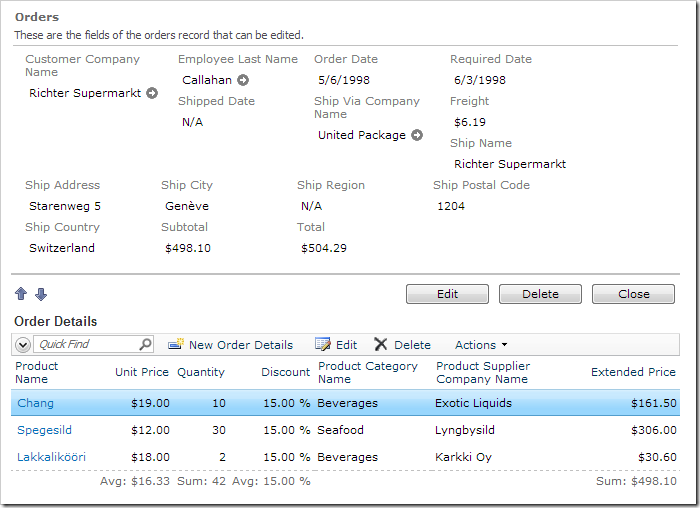Sample Applications / Order Form / Total and Subtotal

# Creating Order Total Field

Sample Applications / Order Form / Total and Subtotal
Creating Order Total Field

Let’s add a Total field to the Orders controller. This field will add Subtotal and Freight together to show the final cost of the whole order.

In the Project Explorer, right-click on Orders / Fields node. Press New Field.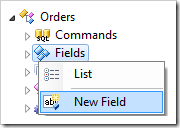Give this new field the following settings:

 Property Value Name Total Type Currency The value of this field is computed at run-time by SQL Expression true SQL Formula ```(select sum(UnitPrice*Quantity*(1-Discount)) from "Order Details" where "Order Details".OrderID = Orders.OrderID) + Orders.Freight ``` The value of this field is calculated by a business rule expression true Label Total Values of this field cannot be edited True Data Format String c Context Fields OrderDetails, Freight

Press OK to save this field.

## Binding the Field to Views

Drop Orders / Fields / Total* (Currency, read-only) field node onto Orders / Views / grid1 node.

Drop Total field node onto Orders / Views / editForm1 view node.

If you used an SQL Business Rule to handle Subtotal, double-click on Orders / Business Rules / Calculate (Sql / Execute) node.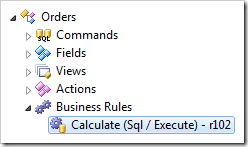Append the following highlighted line to the Script property:

 Property New Value Script ```select @Subtotal = sum(unitprice * quantity * (1 - discount)) from [Order Details] where OrderID = @OrderID set @Total = @Subtotal + @Freight```

If you are using C# or Visual Basic to handle the Subtotal field, right-click on Orders / Business Rules and press Edit Rule in Visual Studio.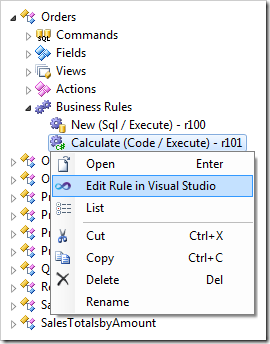Make the following changes to the code base:

C#:

```using System;
using MyCompany.Data;

namespace MyCompany.Rules
{
{

[Rule("r101")]
public void r101Implementation(
int? orderID,
string customerID,
string customerCompanyName,
int? employeeID,
string employeeLastName,
DateTime? orderDate,
DateTime? requiredDate,
DateTime? shippedDate,
int? shipVia,
string shipViaCompanyName,
decimal? freight,
string shipName,
string shipCity,
string shipRegion,
string shipPostalCode,
string shipCountry,
decimal? subtotal)
{
using (SqlText calc = new SqlText(@"select sum(unitprice *
quantity * (1 - discount)) from
[Order Details] where OrderID= @OrderID"))
{
object total = calc.ExecuteScalar();
if (DBNull.Value.Equals(total))
{
UpdateFieldValue("Subtotal", 0);
UpdateFieldValue("Total", 0);
}
else
{
UpdateFieldValue("Subtotal", Convert.ToDecimal(total));
UpdateFieldValue("Total", Convert.ToDecimal(total) + freight);
}
}
}
}
}```

Visual Basic:

```Imports MyCompany.Data
Imports System

Namespace MyCompany.Rules

<Rule("r101")> _
Public Sub r101Implementation( _
ByVal orderID As Nullable(Of Integer), _
ByVal customerID As String, _
ByVal customerCompanyName As String, _
ByVal employeeID As Nullable(Of Integer), _
ByVal employeeLastName As String, _
ByVal orderDate As Nullable(Of DateTime), _
ByVal requiredDate As Nullable(Of DateTime), _
ByVal shippedDate As Nullable(Of DateTime), _
ByVal shipVia As Nullable(Of Integer), _
ByVal shipViaCompanyName As String, _
ByVal freight As Nullable(Of Decimal), _
ByVal shipName As String, _
ByVal shipCity As String, _
ByVal shipRegion As String, _
ByVal shipPostalCode As String, _
ByVal shipCountry As String, _
ByVal subtotal As Nullable(Of Decimal))
Using calc As SqlText = New SqlText(
"select sum(unitprice * quantity * (1 - discount)) " +
"from [Order Details] where OrderID=@OrderID")
Dim total As Object = calc.ExecuteScalar()
If DBNull.Value.Equals(total) Then
UpdateFieldValue("Subtotal", 0)
UpdateFieldValue("Total", 0)
Else
UpdateFieldValue("Subtotal", Convert.ToDecimal(total))
UpdateFieldValue("Total", Convert.ToDecimal(total) + freight)
End If
End Using
End Sub
End Class
End Namespace
```

You can also implement a JavaScript business rule that will use the returned value of Subtotal and sum it with Freight to calculate the value of Total.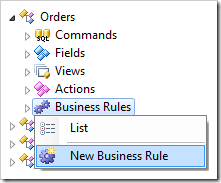Assign the following values:

 Property Value Type JavaScript Command Name Calculate Phase After Script `[Total] = [Subtotal] + [Freight];`

## Viewing the Results

On the toolbar, press Browse to regenerate the application. Navigate to the Order Form page, and select any order. You will see a Total field that sums up Subtotal and Freight.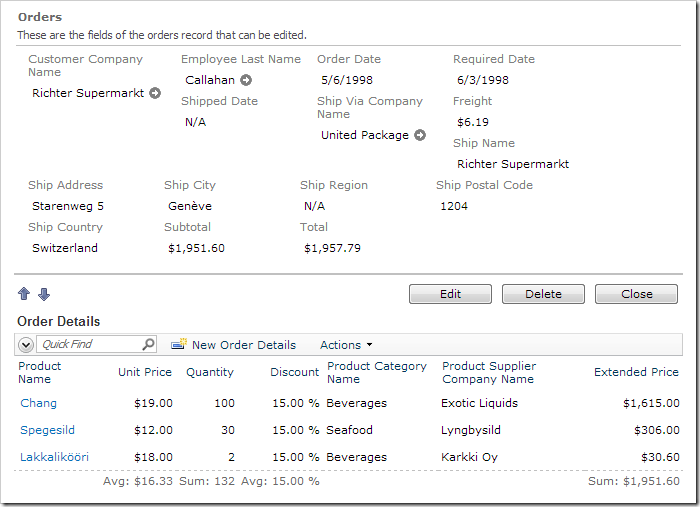When any of the order detail records are changed, the Subtotal and Total will be updated.